Chapter 4 Part 3 - Excess Demand and Excess Supply

Economics Class 12
Macroeconomics

## Decrease of Propensity to Consume (Dec in MPC)

It means consumers start spending less percentage of income

Example

Suppose a person was earning 10000 and earlier spending 7000

Now he is earning same 10000 but he is spending 6000

This less spending leads to lower consumption expenditure and hence less demand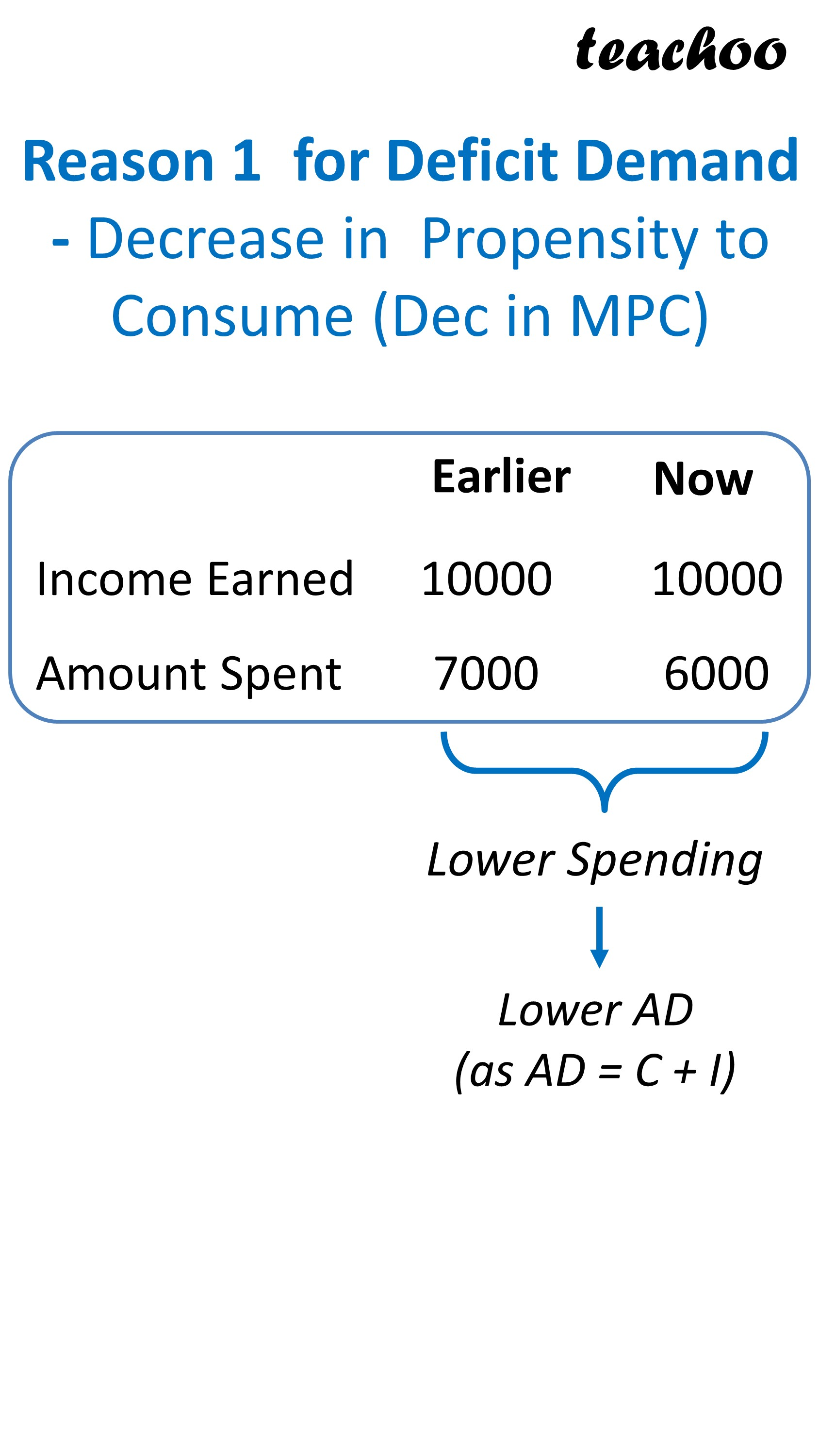## Increase in Taxes

If Tax Rates increase, disposable income decreases, hence person spends less

Example

Suppose a person was earning 50000 and paying tax 10000, his net take home salary (or disposable income) was 500000-10000=40000

Suppose govt reduced taxes to Rs 12000

So now his disposable income became 50000 - 12000=38000

Since, his disposable income decreases by 2000 (40000-38000), he still spend more

This lower spending leads to lower consumption expenditure and hence less demand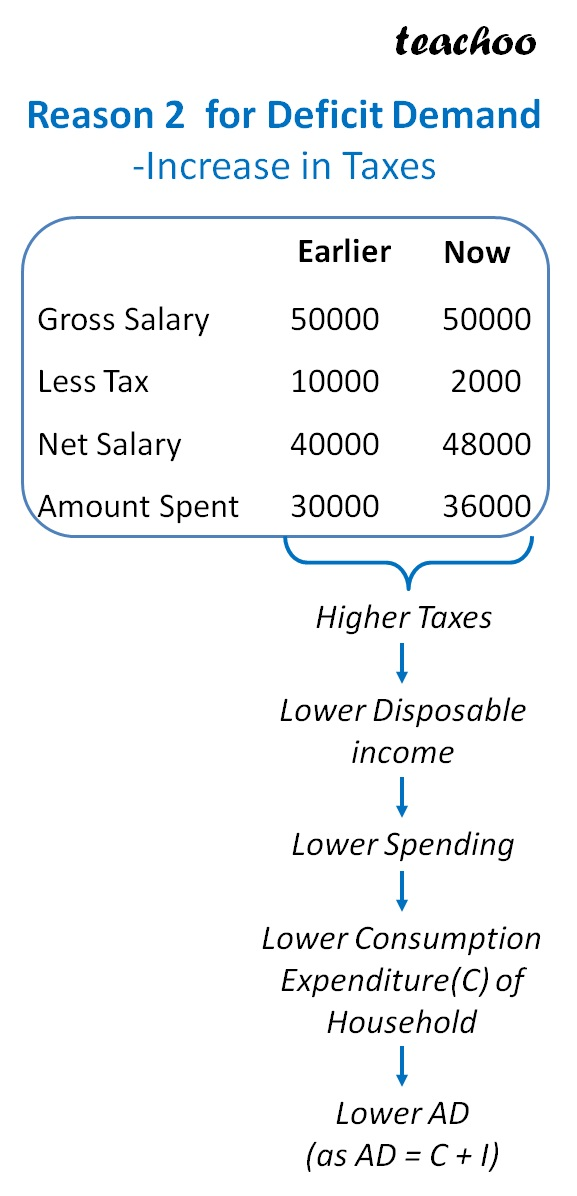## Decrease in Govt Spending

Govt may decrease public expenditure in budget

Hence, govt will be spending less in purchasing goods and service

This lower spending leads to lower consumption expenditure and hence less demand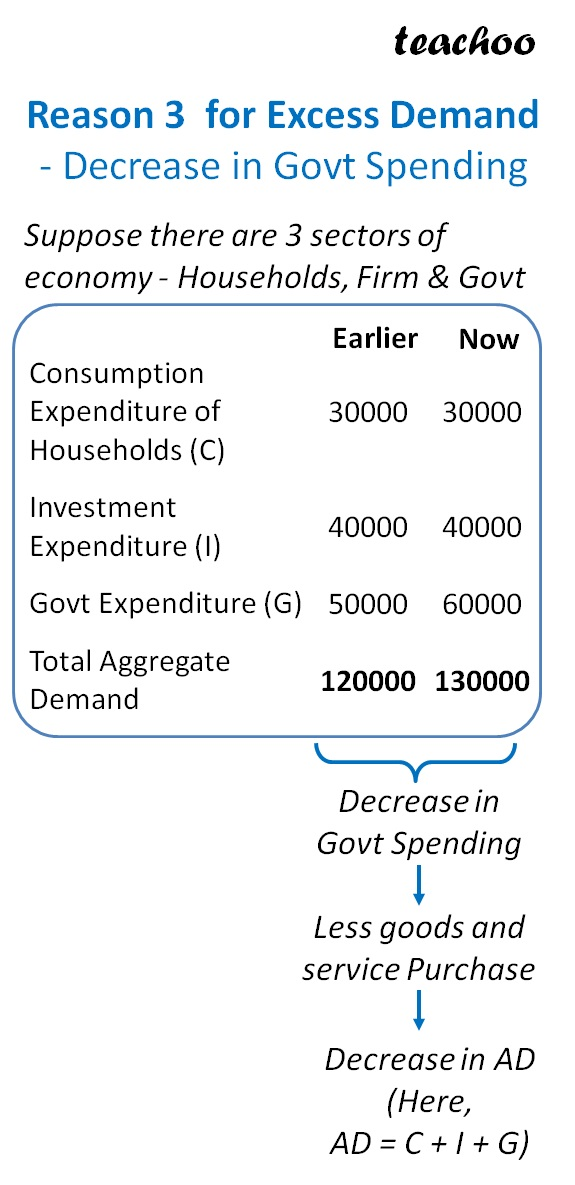## Change in interest Rates

If interest rates on borrowings increase, it will lead to more interest expense and less income

If Int rates on investment decreases, it will lead to less int income

This lower income will lead to less spending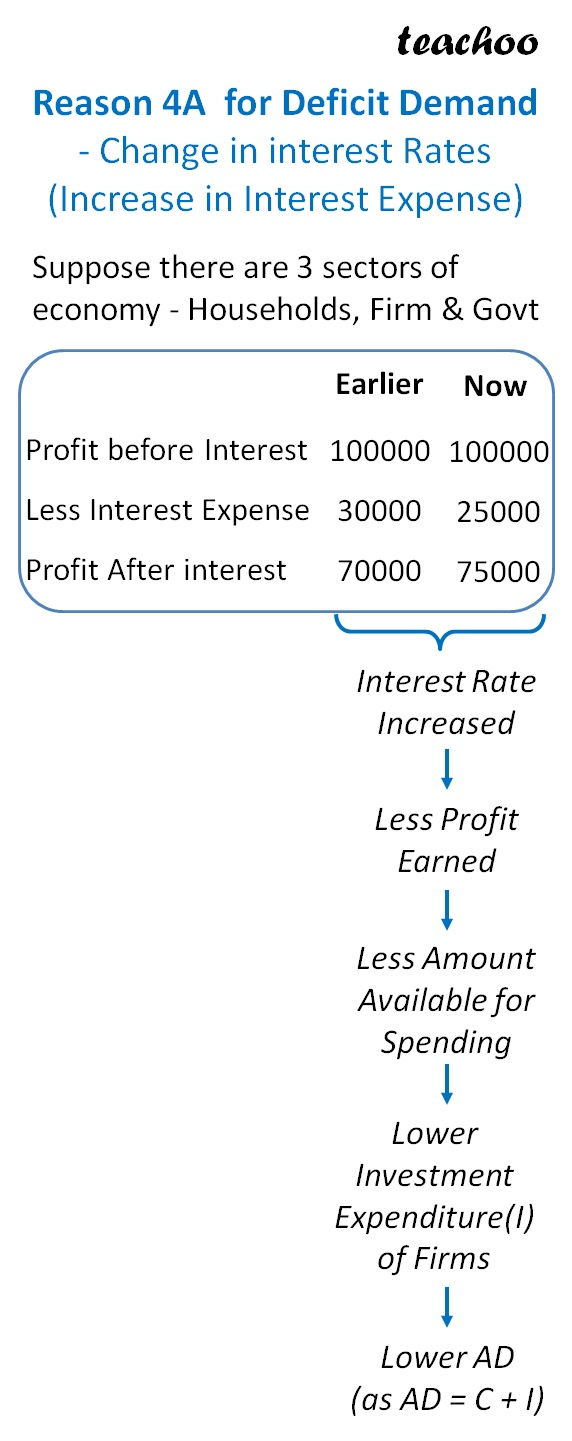## Rise in Import

If we import more goods and services and purchase less from within country

Income of economy will fall

This lower income will lead to less spending

This lower spending leads to lower consumption expenditure and hence less demand

This lower spending leads to lower consumption expenditure and hence less demand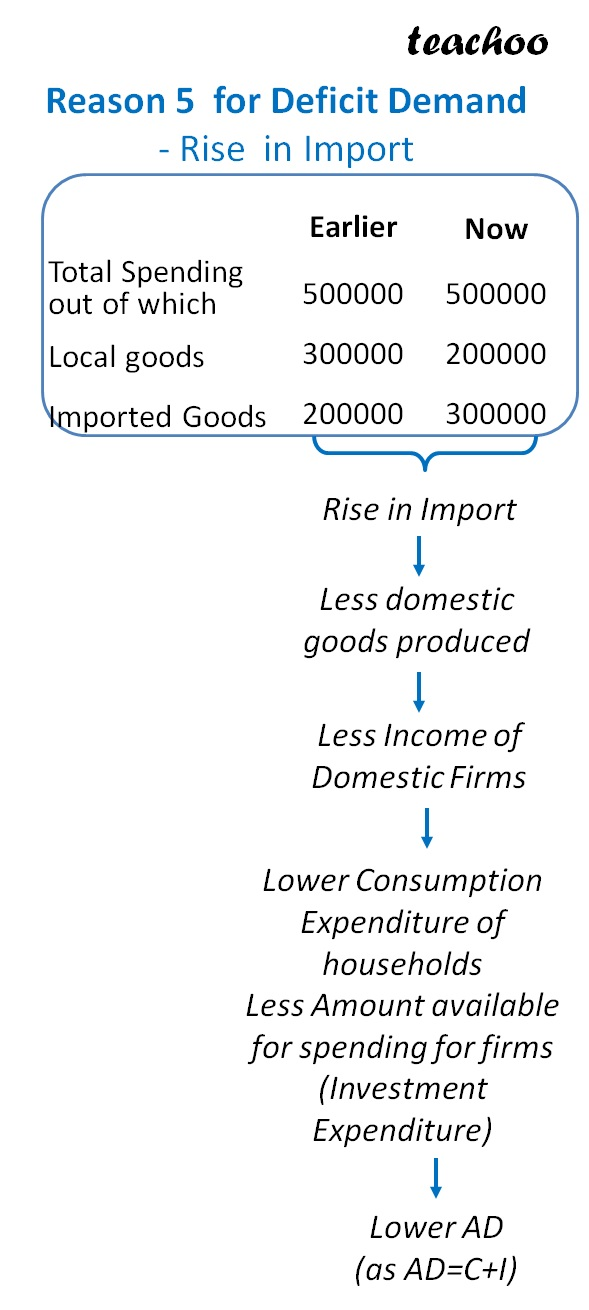## Decrease in Exports

If we export less, it will lead to lower income for the economy

This lower income will lead to less spending

This lower spending leads to lower consumption expenditure and hence less demand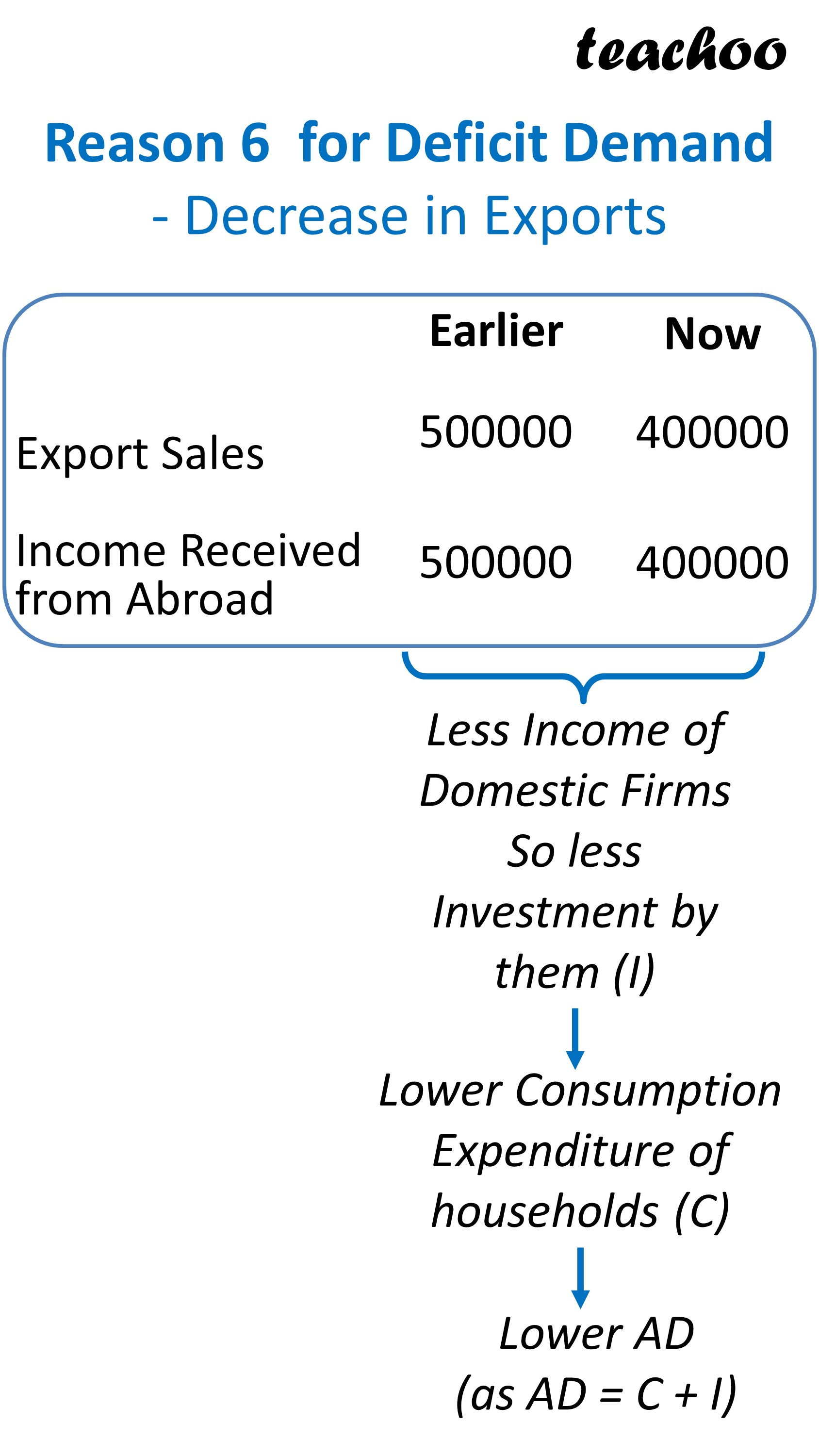### NCERT Questions

No questions in this part

### Other Books

#### Question 1

How does change in Import cause deficit demand?

Learn in your speed, with individual attention - Teachoo Maths 1-on-1 Class

### Transcript

Reason 1 for Deficit Demand - Decrease in Propensity to Consume (Dec in MPC) Income Earned Amount Spent 10000 7000 10000 6000 Lower Spending Lower AD (as AD = C + I) Reason 2 for Deficit Demand -Increase in Taxes Gross Salary Less Tax Net Salary Amount Spent 50000 10000 40000 30000 50000 2000 48000 36000 Higher Taxes Lower Disposable income Lower Spending Lower Consumption Expenditure(C) of Household Lower AD (as AD = C + I) Reason 3 for Excess Demand - Decrease in Govt Spending Suppose there are 3 sectors of economy - Households, Firm & Govt Consumption Expenditure of Households (C) Investment Expenditure (I) Govt Expenditure (G) Total Aggregate Demand 30000 40000 50000 120000 30000 40000 60000 130000 Decrease in Govt Spending Less goods and service Purchase Decrease in AD (Here, AD = C + I + G) Reason 4A for Deficit Demand - Change in interest Rates (Increase in Interest Expense) Suppose there are 3 sectors of economy - Households, Firm & Govt Profit before Interest Less Interest Expense Profit After interest